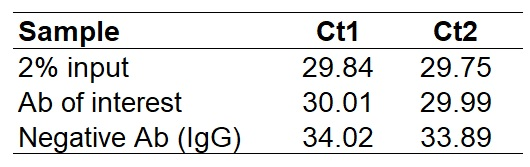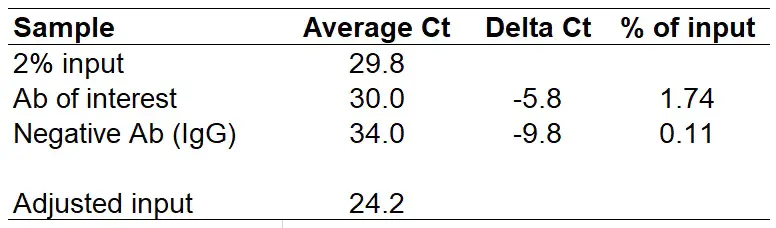# How To Analyse ChIP qPCR Data

There are a few ways in which you can analyse chromatin immunoprecipitation (ChIP) data acquired from quantitative real-time polymerase chain reaction (qPCR). Two of the most common ways to report ChIP qPCR are: percentage of input and fold enrichment.

For the example analysis, I will use the data below. These are qPCR results from a ChIP experiment by using an antibody of interest and a negative (IgG) antibody. The input sample used here is 2%, however, I will explain how to adjust the analysis to accept other input amounts. Instead of the negative IgG antibody sample, you can always replace this with a no antibody sample.

##Percentage of input

The percentage of input analysis represents the amount of DNA pulled down by using the antibody of interest in the ChIP reaction, relative to the amount of starting material (input sample).

### How to calculate the percentage of input

1. After performing the qPCR, firstly average the Ct values for each antibody used.2. Next, we need to adjust the Ct value for the input sample. At the minute this input sample represents 2% of the DNA amount used, therefore we need a value that represents 100% of the DNA amount. To do this, we need to subtract the log of the dilution factor used from the average Ct of the 2% input. In the example we have used a 2% input sample, therefore this is a 50 dilution factor. If a 1% input sample was used, this would be a dilution factor of 100 etc. When log-transforming the data, ensure this is done with a base set at 2. So, in Microsoft Excel the formula would be:

`=LOG(Your dilution factor, 2)`

The ‘2‘ in the formula represents the base in the log calculation, always leave this as ‘2‘.

In the example with a 2% input sample, this would be:

```=LOG(50, 2)
=5.64```

Now, subtract 5.64 from the average Ct of the 2% input to calculate the adjusted input Ct value. Doing this gives a value of ‘24.2‘. I have added this to the results table below.3. The next step is to create delta Ct (i.e. the difference in Ct values between the ‘adjusted input‘ and the antibodies of interest). To do this, simply subtract the Ct values of the antibodies of interest from the ‘adjusted input‘ Ct value. I have done this in a new column called ‘Delta Ct‘.4. Finally, we can calculate the % of input value based on the delta Cts. To do this, we do 2 to the power of ‘Delta Ct‘. Since the results are expressed as a percentage, we also need to multiply this by 100. In Excel, the formula to use is:

`=100*2^(Delta Ct)`

Just replace the Delta Ct with your own delta Ct values. In the above example, we will get the following results:

##Fold enrichment

Fold enrichment presents ChIP results relative to the negative (IgG) sample, in other words the signal over background. The negative sample is given a value of ‘1‘ and everything else will then be a fold change of this negative sample. As opposed to the percentage of input analysis, the fold enrichment does not require an input sample.

### How to calculate the fold enrichment

1. Calculate the delta Ct for the difference between Ct values for the antibody of interest and the negative antibody. To do this, subtract the Ct for the negative antibody from the antibody of interest. Do this for all the samples.Note that the ‘Delta Ct‘ for the negative antibody should always be ‘0‘.

2. To determine the fold enrichment do 2 to the power of negative ‘Delta Ct‘. In Excel, the formula to use is:

`=2^-(Delta Ct)`

Replace ‘Delta Ct‘ with your own delta Ct values. In the above example, we will get the following results:Note that the ‘Fold enrichment‘ for the negative antibody should always be ‘1‘.

## ChIP qPCR analysis Excel template

For those still struggling with the analysis, or just want an easy template to use to quickly calculate the percentage of input and fold enrichment for you, I have created a Microsoft Excel template to freely download. The two different analysis can be found on separate sheets on the file and the instructions are at the top of each sheet.

1.Reggie

Hi Steven,
I just want to thank you for taking time to explain this whole process in very simple terms. Also the excel sheet template is a life saver! Cheers!

2.nancy wang

hi steven
I’d like to know if I could use this method when I analysis the RIP-qPCR the same way
thank you！

•Dr Steven Bradburn, PhD

Hi Nancy,
I have never performed RIP-qPCR, however, I have seen these results commonly presented as % input. So, I presume you can use the same analysis method yes.
Good luck with it 🙂
Steven

3.David

I am performing ChIP QPCR and have calculated the outcome using the Input method described above. However I would like to argue that the ChIP process is many steps (washes, cleanups, etc.) and the pull down amount might not represent the starting input. A better method would be something similar to the Expression delta-delta Ct method (calibrator gene such as GAPDH or beta-Actn is used) where you use instead of a calibrator gene, a negative primer set that targets a gene desert where you know there is not going to be the histone modification or transcription factor present that you are interested in. This primer set would then reflect the actual quantification of DNA pulled down since it would be higher in samples where you had more starting cells and less in samples where you had fewer starting cells. You then just calculate the delta-delta Ct substituting the gene desert CT value where you would put the calibrator CT value.

•Dr Steven Bradburn, PhD

Hi David,
Many thanks for your message and sorry this is a late reply.
I agree with the use of another primer set designed to target a region where the protein of interest will not bind. I always do this in my ChIP experiments to show the enrichment of my primers of interest.
Best wishes,
Steven

4.Dittman

what if I have saved 25uL input, and the remaining 1750uL was evenly distributed into 2 vials, one for target and one for the IgG control go for downstream IP. How should I adjust the Ct value?

•Dr Steven Bradburn, PhD

Hi Dittman,
Many thanks for your comment. So if you have 1750 uL split equally into 2 vials (875 uL each), and 25 ul is used as an input, this represents a 2.857% input sample (25/875*100). In other words this is a 35.00175 dilution factor (100/2.857).
Next, if you do ‘=Log(35.00175, 2)’ in Excel, this gives 5.129. This is the Ct value which needs to be subtracted from your input Ct value to create the adjusted Ct value, ie 100% input sample.
I hope that makes sense.
Steven

5.Fer

Hi, so after collecting input samples crosslinks have to be reversed and DNA precipitation must be performed in them? The volume in which they are resuspended should be the same as the initial volume?
For example, I take 17.5 ul corresponding to 10% of my sample, i must reverse crosslinks and after precipitation resuspend in 17.5 ul? and if I wish to qPCR 1% I must add 1.75 of this sample?
Another question I have is, chiped dna must be resuspended in the same volume of my input sample (17.5) in case of my example and 1.75 added in the qPCR?
Thank you very much!

•Dr Steven Bradburn, PhD

Hi Fer,
Yes, the samples have to be reverse cross-linked. The volumes they are resuspended in doesn’t have to be the same as the initial volume. So long as the volume you resuspend in is the same for all your samples (including the input sample).
I hope that makes sense?
Best wishes,
Steven

6.Meg

I will soon start a ChIP, want to clarify how this 1% and 2% input is calculated? Nothing is explained in the protocol I am using. Is it important to know concentration of Input DNA (after reverse crosslinking) and then decide how much microgram of DNA will be used for ChIP?
If we got total chromatin 330ul, save 30ul as input and use 300ul as ChIP. Then dilute our input 1:50 times before doing qPCR then how the dilution factor for this will be calculated for the adjusted input?
Adjusted input = CT input – LOG((30/300)*50,2)?

•Steven

Hi Meg,